Function Repository Resource:

Perform a folding operation parsing multiple arguments

Contributed by: Sander Huisman

## Examples

### Basic Examples (1)

 In:=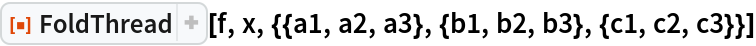Out=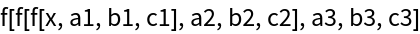### Scope (2)

Without an initializer, the first element of each list is the initializer:

 In:=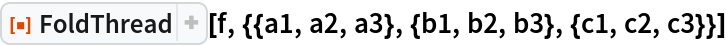Out=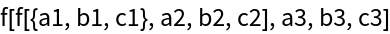Make an operator:

 In:=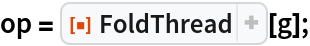Use the operator, feeding in a list of lists:

 In:=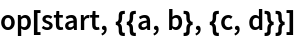Out=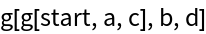### Applications (2)

Integrate the function f(x,y,z)=x+y+z as x ranges from 0 to 2, y from 0 to 2, and z from 0 to 2:

 In:=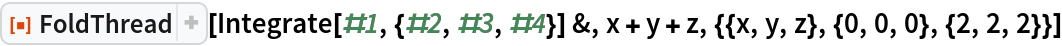Out=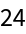Create a continued fraction with different numerators:

 In:=Out=### Properties and Relations (1)

Fold and FoldThread give equivalent results for the case in which a single argument is folded:

 In:=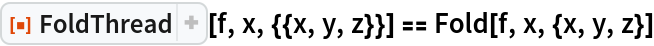Out=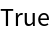SHuisman

## Version History

• 1.0.0 – 17 December 2019